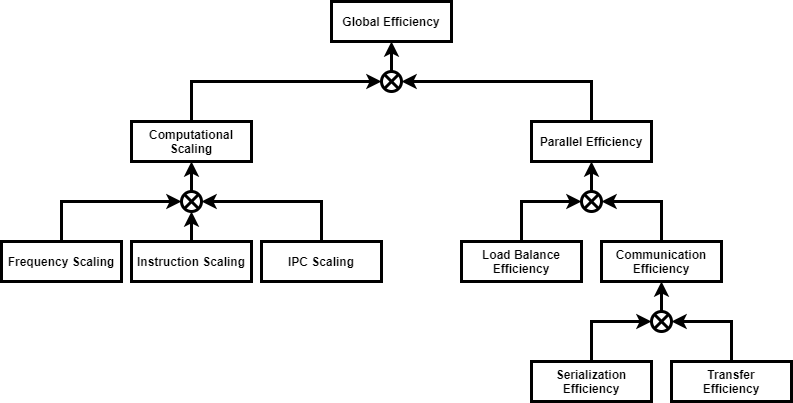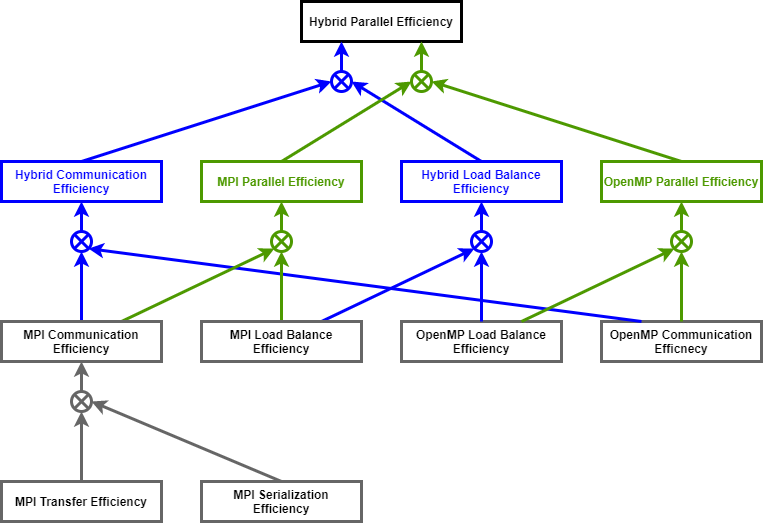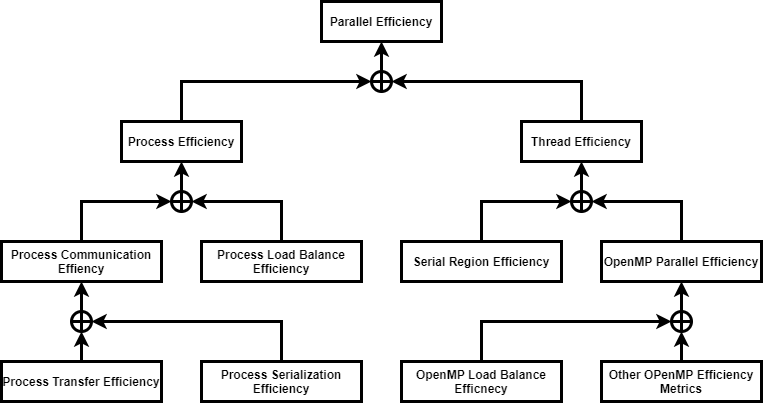# List of Metrics

POP has defined a methodology for analysis of parallel codes to provide a quantitative way of measuring relative impact of the different factors inherent in parallelisation. The the methodology uses a hierarchy of metrics each one reflecting a common cause of inefficiency in parallel programs. These metrics then allow comparison of parallel performance (e.g., over a range of thread/process counts, across different machines, or at different stages of optimisation and tuning) to identify which characteristics of the code contribute to inefficiency.

The metrics are then calculated as efficiencies between 0 and 1, with higher numbers being better. In general, we regard efficiencies above 0.8 as acceptable, whereas lower values indicate performance issues that need to be explored in detail.

### Standard metricsThe Global Efficiency (GE) measures the overall quality of the parallelisation. The Computation Scaling (CompS) computes the ratios of total time in useful computation summed over all processes. The Instruction Scaling (IS) compares total number of useful instructions to the reference case. The IPC Scaling (IPCS) compares IPC to the reference case. The Frequency Scaling (FS) compares processor frequencies to the reference. The Parallel Efficiency (PE) reveals the inefficiency in splitting computation over processes and then communicating data between processes. The Load Balance Efficiency (LBE) is computed as the ratio between Average Useful Computation Time (across all processes) and the Maximum Useful Computation time (also across all processes). The Communication Efficiency (CommE) is the maximum, across all processes, of the ratio between Useful Computation Time and Total Runtime. The Serialisation Efficiency (SerE) describes any loss of efficiency due to dependencies between processes causing alternating processes to wait. The Transfer Efficiency (TE) measures inefficiencies due to time in data transfer.

### Multiplicative Hybrid metricsThe Hybrid Parallel Efficiency (HPE) meassures the percentage of time outside the two parallel runtimes. The Hybrid Communication Efficiency (HCE) is the maximum percentage of time outside both parallel runtimes. The Hybrid Load Balance Efficiency (HLBE) is computed as the ratio between Average Useful Computation Time and the Maximum Useful Computation Time. The MPI Parallel Efficiency (MPI PE) describes the parallel execution of the code in MPI considering that from the MPI point of view, the OpenMP runtime is as useful as the computations. The MPI Load Balance Efficiency (MPI LBE) describes the distribution of work in MPI processes only considering time outside MPI. The MPI Communication Efficiency (MPI CE) describes the loss of efficiency in MPI processes communication only considering time outside MPI. The MPI Serialisation Efficiency (MPI SE) describes any loss of efficiency due to dependencies between processes causing alternating processes to wait. The MPI Transfer Efficiency (MPI TE) measures inefficiencies due to time in data transfer. The OpenMP Parallel Efficiency (OpenMP PE) describes the parallel execution of the code in OpenMP. The OpenMP Communication Efficiency (OpenMP CE) is defined as the quotient of the Hybrid Communication Efficiency (HCE) and the MPI Communication Efficiency (MPI CE) and thereby captures the overhead induced by the OpenMP constructs, which represents threads synchronization and scheduling. The OpenMP Load Balance Efficiency (OpenMP LBE) is defined as the quotient of the Hybrid Load Balance Efficiency (HLBE) and the MPI Load Balance Efficiency (MPI LBE) and represents the load balance within the OpenMP part of the program.